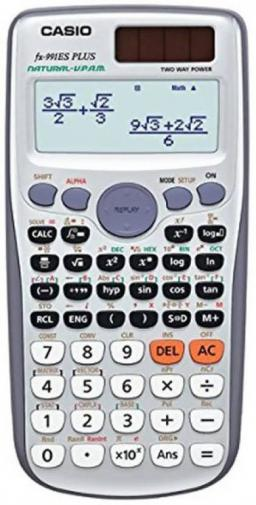# Arithmetic 6299

We enter the number 5068 into the calculator and perform an arithmetic operation, after which the number 2725 appears on display. Which function did we choose?

x =  -2343

### Step-by-step explanation:

5068+x = 2725

x = -2343

x = -2343/1 = -2343

x = -2343

Our simple equation calculator calculates it.Did you find an error or inaccuracy? Feel free to write us. Thank you!